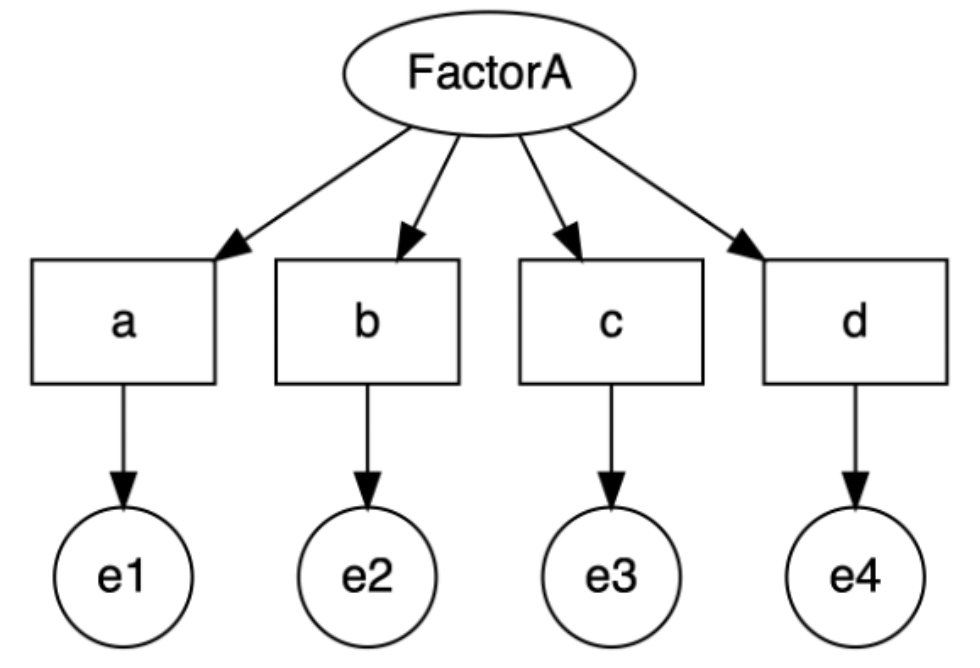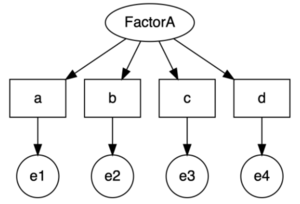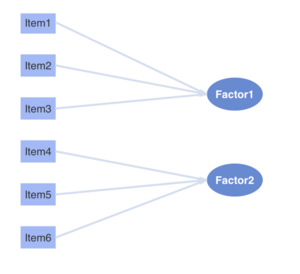# Testing for Measurement Invariance using Lavaan (in R)February 7, 2023

## Testing for Measurement Invariance using Lavaan (in R)### What is Measurement Invariance?

In recent years, there has been an increasing interest in measuring specific unobserved constructs such as individuals’ attitudes, emotions, skills, and perceptions over a wide range of topics–political polarization, student achievement, attitudes towards migration, abortion, etc. To measure these concepts and their different dimensions, researchers often use different strategies to collect self-reported data: they develop a battery of questions or adapt one from other studies. Sometimes, researchers translate surveys into a different language. Whichever their strategy, identifying that their set of questions is measurement invariant is a prerequisite to compare different groups of respondents.

Establishing measurement invariance allows researchers to identify factors that can contribute to a lack of comparability of a scale such as underlying differences between groups (e.g. the same question may have different meaning for different groups); variations in translation of the questions across different languages; use of previously validated scales on populations that are different from the original groups included in the validation sample or inadequate adaptations to the items across different contexts. When we meet the criteria for measurement invariance, we have evidence that our construct has the same meaning across diverse groups of people (Chen, 2007; Putnick & Bornstein, 2016).

Below you will find some basic syntax to test for measurement invariance using lavaan in R. This post is a little bit technical, so if you need some refresher about specific concepts, you can always check a psychometrics book. One I recommend is Recentering Psych Stats Psychometrics by Lynette H. Bikos

### Levels of measurement invariance

Most researchers assess three levels of measurement invariance:

1. Configural invariance: equivalence of the model form

3. Scalar invariance: equivalence of intercepts

A fourth level, strict invariance implies equivalence of the unique variances or item residuals. In practice, meeting the first three levels of invariance, or achieving partial invariance, is considered enough to guarantee appropriate cross-group comparisons of the latent constructs (Van de Schoot et al., 2012). In this blog we describe the steps to test for the first three levels of measurement invariance and the syntax of lavaan, one of the most frequently used functions.

### Testing for Measurement Invariance using Lavaan

The most common approach to test measurement invariance is Multi-Group Confirmatory Factor Analysis (MGCFA). A Confirmatory Factor Analysis (CFA) models the relationship between manifest variables and a latent factor. CFA differs from a similar method known as Exploratory Factor Analysis (EFA) because CFA requires that the relationships between the items and the latent variables are pre-specified whereas EFA is more data-driven and does not necessarily assume a relationship between items.

In R, we can test run a MGCFA using the lavaan and semTools libraries. If you do not have them, you can always install them.

 # Install libraries install.packages(“lavaan”) install.packages(“SemTools”) library(lavaan) library(semTools)

While there are other libraries that can run a MGCFA (e.g. the measurement_invariance command from the psychModel library), lavaan remains one of the most flexible and advanced tools to assess measurement invariance, especially if you are using different types of data (e.g. ordinal or nominal).

Before you start running any estimates, remember to clean your data and define your main CFA model. As a CFA is a Structural Equation Modeling, it has two parts: a measurement model and a structural model. In a simplified manner, you can think of the measurement model part as related to how the latent variables (Factor1 and Factor2) are measured in terms of the observed variables and the structural model as the part that specifies the causal relations among the observed and latent variables (the lines between the items and the latent factors) (Friendly, 2019).This is how we would define this model in lavaan:

 # Two-factor CFA model model<-'Factor1 =~ item1 + item2 + item3        'Factor2 =~ item4 + item5 + item6         Factor1 ~~ Factor2'

Note the use of the syntax:

=~ is used to indicate that a latent factor is connected to an observed variable in the model.

~~ covariance (two items or factors are assumed to be linearly related. For example, higher values of Factor 1 also show higher values of Factor 2).

We now test for configural invariance. In our analyses, we proceed sequentially: we cannot claim metric invariance unless we provide evidence that our model meets the criteria for configural invariance.

 # Configural model cfa.model <- cfa(model, data = mydata, estimator = "WLSMV", group = "mygroup") # We obtain summary statistics using the summary function summary(cfa.model, fit.measures = TRUE, standardized = TRUE)

While there is no consensus yet on the indices and thresholds to determine what a good model is, it is widely acknowledged that Hu & Bentler (1999)’s guidelines are a good starting point to assess the fitness of the model.

Next, to test for configural invariance we add group.equal=’loadings’” to our previous syntax where we defined the configural model

 # Metric model metric.model <- cfa(model,                     data = mydata,                     estimator = "WLSMV",                     group = "mygroup",                      group.equal= “loadings”) # We can compare models using compareFit compareFit(cfa.model, cfa.metric)

Finally, to test for scalar invariance, we add intercepts to the list of parameters that we need to constrain and we make them equal across the different groups in our dataset.

 # Scalar model cfa.scalar <- cfa(model,                   data=mydata,                   estimator = "WLSMV",                   group = "mygroup",                    group.equal = c(“loadings”, “intercepts”)) # Compare the models compareFit(cfa.metric, cfa.scalar)

A list of relevant statistics will allow you to examine how appropriate the scalar model is and whether measurement invariance was achieved.

### Some final remarks…

While obtaining partial invariance (configural, metric and scalar invariance) still allows you to compare the groups in the latent constructs, high quality (and high-stakes) instruments should aim to meet the strict invariance level.

### References

Chen, F. (2007). Sensitivity of Goodness of Fit Indexes to Lack of Measurement Invariance. Structural Equation Modeling: A Multidisciplinary Journal 14(3). doi:10.1080/10705510701301834

Friendly, M. (2019). Confirmatory Factor Analysis & Structural Equation Models Lecture 1: Overview & Path Analysis. Retrieved from: https://www.datavis.ca/courses/CFA-SEM/lectures/CFA-SEM1-4up.pdf

Hu, L. & Bentler, P. (2012). Cutoff criteria for fit indexes in covariance structure analysis: Conventional criteria versus new alternatives. Structural Equation Modeling: A Multidisciplinary Journal 6. Doi: 10.1080/10705519909540118

Putnick, D. & Bornstein, M. (2016) Measurement Invariance Conventions and Reporting: The State of the Art and Future Directions for Psychological Research. Dev. Rev. 41. doi:10.1016/j.dr.2016.06.004

Van de Schoot, R., Lugtig, P. & Hox, J. (2012). A checklist for testing measurement invariance. European Journal of Developmental Psychology. 9(4). doi:10.1080/17405629.2012.686740